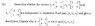# Can a matrix be a vector?

judahs_lion
This question makes no since to me are these matrixes v1, v2 ,v3 actually vectors as stated in the problem attached?

#### Attachments

•takehomeexam10.jpg
16.1 KB · Views: 374

Homework Helper
They are not vectors in the form that you usually encounter them (i.e. as a string of coordinates in n-dimensional space).
However, if you call any element of a vector space (that is, a set that satisfies certain axioms) a vector, then indeed they are.

Note by the way that there is always a bijection between n x m matrices and vectors in Rnm.

judahs_lion
How would I go about finding the determinate of them or would I have to go about another method to test for independence?

I can tell the vector {4,4;4,4} would not be in the span because there is no multiple of d for({a, b;c,d} that would ever equal 4 because d is always 0.

Staff Emeritus
You don't need the determinant to solve this problem. You are just looking for some a, b such that $a\,\mathbf v_1 + b\,\mathbf v_2 = \mathbf v_3$ -- or show that no such a, b exist.

judahs_lion
You don't need the determinant to solve this problem. You are just looking for some a, b such that $a\,\mathbf v_1 + b\,\mathbf v_2 = \mathbf v_3$ -- or show that no such a, b exist.

Thanks so a = -7, b =1;

Span(v1,v2,v3) = {<a, -a; c , 0> : a, c belong to R} ?

Staff Emeritus
Gold Member
Thanks so a = -7, b =1;
Nope. Edit: Oops, my bad. I checked it again after seeing DH's post, and I'm the one who messed up.

I suggest you use the standard definition of linear independence. $\{v_1,\dots,v_k\}$ is linearly independent if

$$\sum a_i v_i=0\Rightarrow a_i=0 \text{ for all }i$$

(What DH said works too).

Last edited:
Staff Emeritus
Thanks so a = -7, b =1;
Good. So what does that mean in terms of the problem? (You need to determine whether v1, v2, v3 are dependent or independent.)

Span(v1,v2,v3) = {<a, -a; c , 0> : a, c belong to R} ?
That looks good.

judahs_lion
Good. So what does that mean in terms of the problem? (You need to determine whether v1, v2, v3 are dependent or independent.)

That looks good.

That means they are dependent? v3 depends on v1 and v2

Staff Emeritus